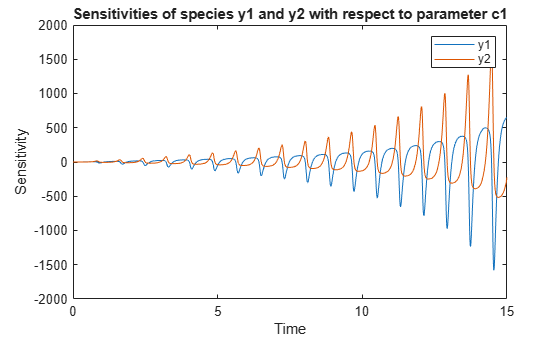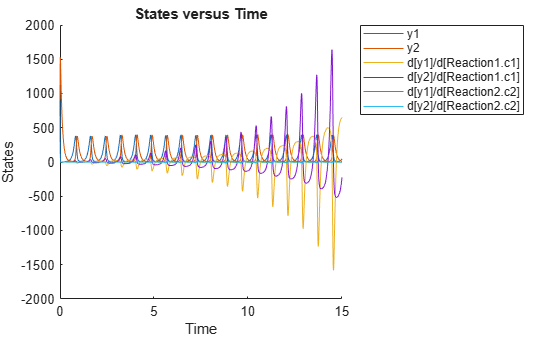# SimFunctionSensitivity object

SimFunctionSensitivity object, subclass of `SimFunction` object

## Description

The `SimFunctionSensitivity` object is a subclass of `SimFunction object`. It allows you to compute sensitivity.

## Syntax

The `SimFunctionSensitivity` object shares all syntaxes of the `SimFunction` object. It has the following additional syntax.

`[T,Y,SensMatrix] = F(___)` returns `T`, a cell array of numeric vector, `Y`, a cell array of 2-D numeric matrices, and `SensMatrix`, a cell array of 3-D numeric matrix containing calculated sensitivities of model quantities. `SensMatrix` contains a matrix of size TimePoints x Outputs x Inputs. TimePoints is the total number of time points, Outputs is the total number of output factors, and Inputs is the total number of input factors.

If you specify a single output argument, the object returns an `SimData` object or array of `SimData` objects with sensitivity information.

## Properties

The `SimFunctionSensitivity` object shares all properties of the `SimFunction object`. It has the following additional properties.

 `SensitivityOutputs` `table` with variables named: `'Name'``'Type'``'Units'` (only if `UnitConversion` is turned on)This table contains information about model quantities (species or parameters) for which you want to compute the sensitivities. Sensitivity output factors are the numerators of time-dependent derivatives described in Sensitivity Analysis in SimBiology. This property is read only. `SensitivityInputs` `table` with variables named: `'Name'``'Type'``'Units'` (only if `UnitConversion` is turned on)This table contains information about model quantities (species, compartments, or parameters) with respect to which you want to compute the sensitivities. Sensitivity input factors are the denominators of time-dependent derivatives described in Sensitivity Analysis in SimBiology. This property is read only. `SensitivityNormalization` Character vector specifying the normalization method for calculated sensitivities. The following examples show how sensitivities of a species x with respect to a parameter k are calculated for each normalization type.`'None'` — No normalization. `$\frac{\partial x\left(t\right)}{\partial k}$``'Half'` — Normalization relative to the numerator only. `$\left(\frac{1}{x\left(t\right)}\right)\left(\frac{\partial x\left(t\right)}{\partial k}\right)$``'Full'` — Full dedimensionalization `$\left(\frac{k}{x\left(t\right)}\right)\left(\frac{\partial x\left(t\right)}{\partial k}\right)$`

## Examples

collapse all

This example shows how to calculate the sensitivities of some species in the Lotka-Volterra model using the `SimFunctionSensitivity` object.

`sbioloadproject lotka;`

Define the input parameters.

`params = {'Reaction1.c1', 'Reaction2.c2'};`

Define the observed species, which are the outputs of simulation.

`observables = {'y1', 'y2'};`

Create a `SimFunctionSensitivity` object. Set the sensitivity output factors to all species (`y1` and `y2`) specified in the `observables` argument and input factors to those in the `params` argument (`c1` and `c2`) by setting the name-value pair argument to `'all'`.

`f = createSimFunction(m1,params,observables,[],'SensitivityOutputs','all','SensitivityInputs','all','SensitivityNormalization','Full')`
```f = SimFunction Parameters: Name Value Type ________________ _____ _____________ {'Reaction1.c1'} 10 {'parameter'} {'Reaction2.c2'} 0.01 {'parameter'} Observables: Name Type ______ ___________ {'y1'} {'species'} {'y2'} {'species'} Dosed: None Sensitivity Input Factors: Name Type ________________ _____________ {'Reaction1.c1'} {'parameter'} {'Reaction2.c2'} {'parameter'} Sensitivity Output Factors: Name Type ______ ___________ {'y1'} {'species'} {'y2'} {'species'} Sensitivity Normalization: Full ```

Calculate sensitivities by executing the object with `c1` and `c2` set to 10 and 0.1, respectively. Set the output times from 1 to 10. `t` contains time points, `y` contains simulation data, and `sensMatrix` is the sensitivity matrix containing sensitivities of `y1` and `y2` with respect to `c1` and `c2`.

`[t,y,sensMatrix] = f([10,0.1],[],[],1:10);`

Retrieve the sensitivity information at time point 5.

```temp = sensMatrix{:}; sensMatrix2 = temp(t{:}==5,:,:); sensMatrix2 = squeeze(sensMatrix2)```
```sensMatrix2 = 2×2 37.6987 -6.8447 -40.2791 5.8225 ```

The rows of `sensMatrix2` represent the output factors (`y1` and `y2`). The columns represent the input factors (`c1` and `c2`).

`$sensMatrix2=\left[\begin{array}{cc}\begin{array}{c}\frac{\partial y1}{\partial c1}\\ \\ \frac{\partial y2}{\partial c1}\end{array}& \begin{array}{c}\frac{\partial y1}{\partial c2}\\ \\ \frac{\partial y2}{\partial c2}\end{array}\end{array}\right]$`

Set the stop time to 15, without specifying the output times. In this case, the output times are the solver time points by default.

`sd = f([10,0.1],15);`

Retrieve the calculated sensitivities from the `SimData` object `sd`.

`[t,y,outputs,inputs] = getsensmatrix(sd);`

Plot the sensitivities of species `y1` and `y2` with respect to `c1`.

```figure; plot(t,y(:,:,1)); legend(outputs); title('Sensitivities of species y1 and y2 with respect to parameter c1'); xlabel('Time'); ylabel('Sensitivity');```Plot the sensitivities of species `y1` and `y2` with respect to `c2`.

```figure; plot(t,y(:,:,2)); legend(outputs); title('Sensitivities of species y1 and y2 with respect to parameter c2'); xlabel('Time'); ylabel('Sensitivity');```Alternatively, you can use `sbioplot`.

`sbioplot(sd);`You can also plot the sensitivity matrix using the time integral for the calculated sensitivities of `y1` and `y2`. The plot indicates `y1` and `y2` are more sensitive to `c1` than `c2`.

```[~, in, out] = size(y); result = zeros(in, out); for i = 1:in for j = 1:out result(i,j) = trapz(t(:),abs(y(:,i,j))); end end figure; hbar = bar(result); haxes = hbar(1).Parent; haxes.XTick = 1:length(outputs); haxes.XTickLabel = outputs; legend(inputs,'Location','NorthEastOutside'); ylabel('Sensitivity');```### Topics

Introduced in R2015a

## SupportGet trial now